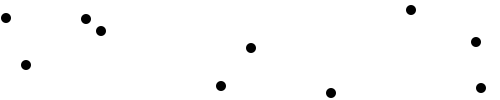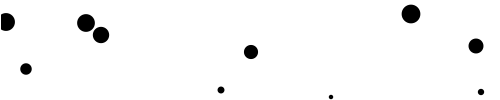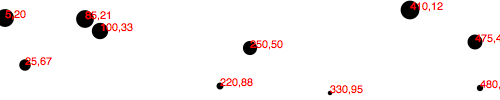# Making a scatterplot

So far, we’ve drawn only bar charts with simple data — just one-dimensional sets of numbers.

But when you have two sets of values to plot against each other, you need a second dimension. The scatterplot is a common type of visualization that represents two sets of corresponding values on two different axes: horizontal and vertical, x and y.

## The Data

As you saw in Types of data, you have a lot of flexibility around how you structure your data set. For our scatterplot, I’m going to use an array of arrays. The primary array will contain one element for each data “point.” Each of those “point” elements will be another array, with just two values: one for the x value, and one for y.

```var dataset = [

[5, 20], [480, 90], [250, 50], [100, 33], [330, 95],
[410, 12], [475, 44], [25, 67], [85, 21], [220, 88]
];

```

Remember, `[]` means array, so nested hard brackets `[[]]` indicate an array within another array. We separate array elements with commas, so an array containing three other arrays would look like: `[[],[],[]]`

We could rewrite our data set so it’s easier to read, like so:

```var dataset = [

[ 5,     20 ],
[ 480,   90 ],
[ 250,   50 ],
[ 100,   33 ],
[ 330,   95 ],
[ 410,   12 ],
[ 475,   44 ],
[ 25,    67 ],
[ 85,    21 ],
[ 220,   88 ]
];

```

Now you can see that each of these 10 rows will correspond to one point in our visualization. With the row `[5, 20]`, for example, we’ll use `5` as the x value, and `20` for the y.

## The Scatterplot

Let’s carry over most of the code from our bar chart experiments, including the piece that creates the SVG element:

```//Create SVG element

var svg = d3.select("body")
.append("svg")
.attr("width", w)
.attr("height", h);

```

Instead of creating `rect`s, however, we’ll make a `circle` for each data point:

```svg.selectAll("circle")

.data(dataset)
.join("circle")

```

Also, instead of specifying the `rect` attributes of `x`, `y`, `width`, and `height`, our `circle`s need `cx`, `cy`, and `r`:

```   .attr("cx", function(d) {

return d;
})
.attr("cy", function(d) {
return d;
})
.attr("r", 5);

```Here’s that working scatterplot.

Notice how we access the data values and use them for the `cx` and `cy` values. When using `function(d)`, D3 automatically hands off the current data value as `d` to your function. In this case, the current data value is one of the small arrays in our larger `dataset` array.

When `d` is an array of values (and not just a single value, like `3.14159`), you need to use bracket notation to access its values. Hence, instead of `return d`, we have `return d` and `return d`, which return the first and second values of the array, respectively.

For example, in the case of our first data point `[5, 20]`, the first value (array position `0`) is `5`, and the second value (array position `1`) is `20`. Thus:

```d returns 5

d returns 20

```

By the way, if you ever want to access any value in the larger data set (outside of D3, say), you can do so using bracket notation. For example:

```dataset returns [410, 12]

```

You can string brackets together, to access values within nested arrays:

```dataset returns 12

```

Don’t believe me? Take another look at the scatterplot, open your JavaScript console, and try typing in `dataset` or `dataset` and see what happens.

## Size

Maybe you want the circles to be different sizes, so their radii correspond to their y values. Instead of setting all `r` values to `5`, try:

```

.attr("r", function(d) {

return Math.sqrt(h - d);
});

```This is neither pretty nor useful, but it illustrates how you might use `d`, along with bracket notation, to reference data values and set `r` accordingly.

## Labels

Let’s label our data points with `text` elements. I’ll adapt the label code from our bar chart experiments, starting with:

```

svg.selectAll("text")

.data(dataset)
.join("text")

```

So far, this looks for all `text` elements in the SVG (there aren’t any yet), and then appends a new `text` element for each data point. Use the `text()` method to specify each element’s contents:

```

.text(function(d) {

return d + "," + d;
})

```

This looks messy, but bear with me. Once again, we’re using `function(d)` to access each data point. Then, within the function, we’re using both `d` and `d` to get both values within that data point array.

The plus `+` symbols, when used with strings, such as the comma between quotation marks `","`, act as append operators. So what this one line of code is really saying is: Get the values of `d` and `d` and smush them together with a comma in the middle. The end result should be something like `5,20` or `25,67`.

Next, we specify where the text should be placed with `x` and `y` values. For now, let’s just use `d` and `d`, the same values that we used to specify the `circle` positions.

```

.attr("x", function(d) {

return d;
})
.attr("y", function(d) {
return d;
})

```

Finally, add a bit of font styling with:

```

.attr("font-family", "sans-serif")

.attr("font-size", "11px")
.attr("fill", "red");

```Here’s that working code.

## Next Steps

Hopefully, some core concepts of D3 are becoming clear: Loading data, generating new elements, and using data values to derive attribute values for those elements.

Yet the image above is barely passable as a data visualization. The scatterplot is hard to read, and the code doesn’t use our data flexibly. To be honest, we haven’t yet improved on — gag — Excel’s Chart Wizard!

Not to worry: D3 is way cooler than Chart Wizard (not to mention Clippy), but generating a shiny, interactive chart involves taking our D3 skills to the next level. To use data flexibly, we’ll learn about D3’s scales. To make our scatterplot easier to read, we’ll learn about axis generators and axis labels. Following that, we’ll make things interactive and learn how to update data on the fly.

Next up: Scales